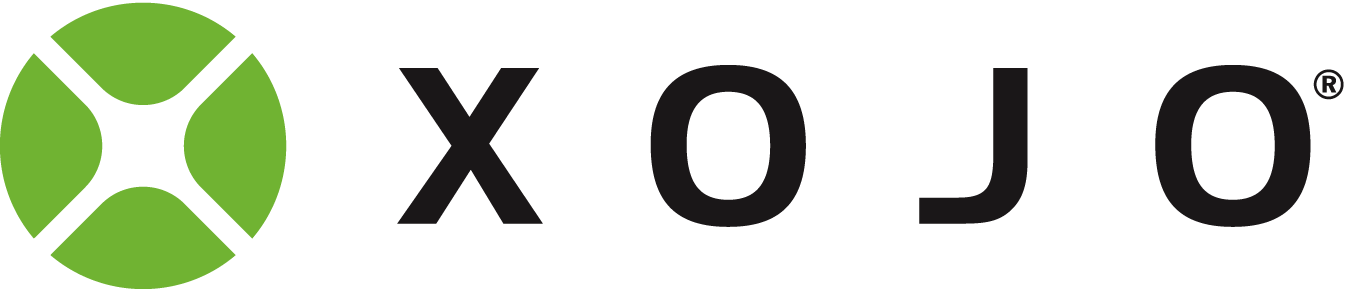Drawing spirals

Anybody has the code to draw a (Archimedes) spiral on canvas?

Rosetta Code is a great resource for stuff like this. Look here for a starting point.

https://rosettacode.org/wiki/Archimedean_spiral

2 Likes

Wow! It is.

Thanks Kem.

I see REALBasic is listed, but Xojo isnt, at https://rosettacode.org/wiki/Category:Programming_Languages

Read more carefully… the 11th entry below X…

https://rosettacode.org/wiki/Category:Xojo

The first language on that page is Action! … That is a real bast from the past!!!

I used that on my Atari 800 back in the 1980’s.

-Karen

I used the QBasic code listed:

SCREEN 12
WINDOW (-2.67, -2!)-(2.67, 2!)
PI = 4 * ATN(1)
H = PI / 40
A = .2: B = .05
PSET (A, 0)
FOR I = 0 TO 400
T = I * H
X = (A + B * T) * COS(T)
Y = (A + B * T) * SIN(T)
LINE -(X, Y)
NEXT

and translated it into Xojo method which takes some canvas dimensions as parameters

Public Sub DrawArchimedeanSpiral(CanvasWidth as Integer, CanvasHeight as Integer)
'Xojo code to draw an Archimedan Spiral
'Alexander van der Linden

'Create a picture

Var SpiralPicture As New Picture(CanvasWidth,CanvasHeight)

'call the graphics class
Var G As Graphics

'the spiral algorithm draws a line between two points
'the -old- X and Y coordinates from the previous loop
'and the new calculated coordinates in the current loop

'create vars
Var PrevX,PrevY As Double
Var X, Y As Double

'center the starting point
X = CanvasWidth/2
Y = CanvasHeight/2

PrevX = X
PrevY = Y

'calculate Pi
Var Pi As Double = 4 * ATan(1)

'these vars determine distance of the lines

Var H As Double = Pi / 40
Var A As Double = 0.1
Var B As Double = 0.1
Var T As Double

'play with I to size the spiral

For I As Integer = 0 To 4000
T = I * H

'I added the X and Y to centre the picture as
'the original algorithm uses a different coordinate system
X = (A + B * T) * Cos(T) + X
Y = (A + B * T) * Sin(T) + Y

'draw the line
SpiralPicture.Graphics.DrawLine(PrevX,PrevY,X,Y)

'store for next loop
PrevX = X
PrevY = Y

Next

'show it on the canvas
PlayCanvas.Backdrop = SpiralPicture
End Sub

2 Likes
Forum for Xojo Programming Language and IDE. Copyright © 2021 Xojo, Inc.